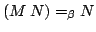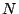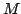Next: Generation of fixpoint using Up: Basic Usage of Y-Combinator Previous: Basic Usage of Y-Combinator   Contents   Start

#### Fixpoint of a Function

If it can be shown thatis true, thenis called either a fixed point' or a fixpoint' of.

According to H. Curry, there exists a function, that generates such a fixed point of. This function is the so-called Y-combinator introduced above.According to the above definition a fixpoint of a function can be seen as a lambda expression that, if passed as argument to this function is returned by the function.

Georg P. Loczewski 2004-03-05

Impressum und Datenschutz